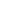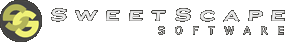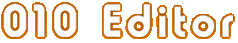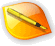Edit AnythingProfessional text and hex editingwith Binary Templates technology.010 Editor - Text/Hex Editor Homepage

 Introduction to Number SystemsWhen editing raw hex data, 010 Editor uses a variety of different number systems including decimal, hexadecimal, octal, and binary. Each number system has a different 'base' that is used to convert from a set of digits to a numeric value. For example, the digits '246' can be converted to a number using base 10 by 2*102 + 4*10 + 6 = 246. In general, if the n digits of a number A are numbered where A0 is the right-most digit, A1 is the digit to the left and so on, then the value of a number of base B is calculated:

An-1*Bn-1 + An-2*Bn-2 + ... + A1*B + A0

The following is a list of the 4 number systems used:

• Decimal - Numbers are represented as base 10. The digits may be any number from '0' to '9'. For example, in decimal 153 = 1*102 + 5*101 + 3.

• Hexadecimal - Numbers are represented as base 16. All the decimal digits are used, plus the letters 'A', 'B', 'C', 'D', 'E', and 'F' are used to represent the numbers 10 through 15. For example, in hexadecimal 3d7 = 3*162 + 13*161 + 7 = 983. This system is commonly referred to as Hex.

• Octal - Numbers are represented as base 8. Only the digits '0' through '7' are used ('8' or '9' is not allowed). For example, the number 2740 = 2*83 + 7*82 + 4*81 + 0 = 1504.

• Binary - Numbers are represented as base 2. Only the digits '0' or '1' can be used. For example, the number 10110 = 1*24 + 0*23 + 1*22 + 1*21 + 0 = 22.## Bits and Bytes

A digit of a binary number is also called a 'bit'. When 8 bits are grouped together, the result is called a 'byte'. Since a byte has 8 binary digits, it can represent any value from 0 up to 255 inclusive. Every file stored on a disk is stored as a set of bytes. Note that when 4 bits are grouped together (base 2), this can also be represented as a single hexadecimal digit (base 16). For example, binary 0101 = '5' hexadecimal, or binary 1111 = 'F' hexadecimal.

010 Editor is designed specifically for editing the individual bytes of a file. When a file is opened for editing, a Hex Editor Window shows the representation of each byte as a hexadecimal number and as a character (see Introduction to Editing for more information).## Entering Numbers in Text Fields

Almost anywhere a number is entered in 010 Editor (in most text fields, the Inspector, etc.), the program supports input in a number of different formats. Usually, the format of the number is assumed to be decimal (some fields have a Decimal and Hex toggle beside them - clicking the Hex toggle will set the default to be hex). However, the other formats can be entered with a special syntax:

• Hex - Use '0x' before the number or ',h', ',x', or 'h' after the number. For example, '0x100' or '3f,h', 'd2,x', or 'FFh' represent hexadecimal numbers.

• Octal - Enter ',o' after the number. For example, '377,o' is an octal number.

• Binary - Enter ',b' after the number. For example, '0101,b' is a binary number.

• Decimal - Enter ',d' after the number. For example, '123,d' is a decimal number.

• Characters - Characters can be entered by placing single quotes around the character. For example, 'A' would be converted to the number 65. Most standard C escape sequences are also supported using '\'. For example, '\n' would be converted to the number 10.

See Introduction to Byte Ordering for information on how to group bytes together to make numbers larger than 255.

The number formats supported in Scripts and Templates are slightly different. See Introduction to Templates and Scripts for more information.010 Editor v14.0 Manual - Windows EditionCopyright © 2003-2023 SweetScape Software - www.sweetscape.com

This is the manual for 010 Editor, a professional hex editor and text editor. Use 010 Editor to edit the individual bytes of any binary file, hard drive, or process on your machine. 010 Editor contains a whole host of powerful analysis and editing tools, plus Binary Templates technology that allows any binary format to be understood.Newsletter - Receive special offers, tips, tricks and news. Join now010 Editor v14.0 is here! What's new?NavigationProducts010 Editor